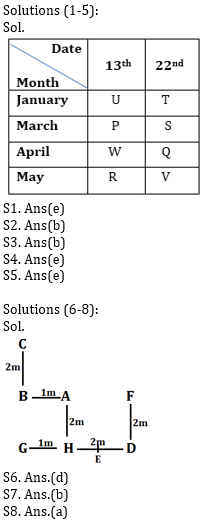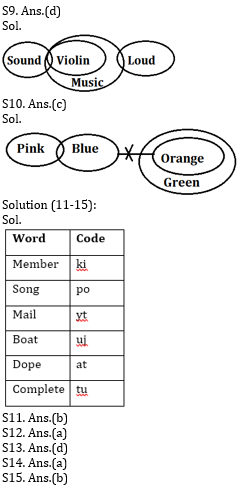# Reasoning Ability Quiz for Prelims Exams- SBI & IBPS 2020- 5th December

Directions (1-5): Study the information carefully and answer the questions given below.

Eight persons P, Q, R, S, T, U, V and W are going for holidays on two different dates (13th and 22nd) in four different months i.e. January, March, April and May. All information is not necessarily in same order.
Q goes in April. Three person goes between Q and T. Equal number of persons goes after T and Before R. More than one person goes between R and S who does not goes on odd number date. One person goes between P and W. Equal number of persons goes after V and Before U who goes on odd number date. P does not go in the month of Q.

Q1. Who among the following person goes on 13th January?
(a) V
(b) T
(c) S
(d) Q
(e) None of these

Q2. How many persons goes between V and S?
(a) One
(b) Three
(c) Four
(d) Five
(e) None of these

Q3. Who among the following person goes on even number date of the month which has even number of days?
(a) W
(b) Q
(c) U
(d) V
(e) None of these

Q4. How many persons go before S?
(a) Two
(b) One
(c) Four
(d) Five
(e) None of these

Q5. Which of the following combination is true?
(a)13th January-Q
(b) 22nd April-R
(c) 13th April-V
(d) 22nd March-P
(e) None is true

Directions (6-8): Study the information given below carefully and answer the questions that follow:
Point C is 2 m north of Point B. Point A is 1 m east of B and Point H is 2 m south of A. Point G is 1 m west of point H while Point D is 3 m east of point G and Point F is 2 m north of point D. Point E is just in middle of point H and point D.

Q6. If Point K is 2m north of point F, then what is the shortest distance between point G to point K?
(a) 8m
(b) 7m
(c) 10m
(d) 5m
(e) None of these

Q7. In which direction is point C with respect to D?
(a) North-East
(b) North-West
(c) South-East
(d) West
(e) None of these

Q8. What is the distance and direction of point B with respect to F?
(a) 3m, West
(b) 5m, North
(c) 7m, South
(d) 3m, East
(e) None of these

Directions (9-10): In each question below are given some statements followed by some conclusions. You have to take the given statements to be true even if they seem to be at variance with commonly known facts. Read all the conclusions and then decide which of the given conclusions logically follows from the given statements, disregarding commonly known facts.

Q9. Statements:
I. Some sound are violin.
II. All violin are music.
III. only few music are loud.
Conclusions:
I. Some loud are sound is a possibility
II. No violin are loud.
III. Some music are sound.
(a) Only I follows
(b) Only III follows
(c) Both I and II follows
(d) Both I and III follows
(e) None of these

Q10. Statements:
I. Only a few pink are blue.
II. No blue are orange.
III. All orange are green.
Conclusions:
I. No orange is pink
II. Some Green is blue is a possibility
III. All pink is blue is a possibility
(a) Only III follows
(b) Both I and II follow
(c) Only II
(d) Both II and III follows
(e) None of these

Directions (11-15): Study the following information carefully and answer the questions given below:
In a certain code language:
‘member song mail’ is written as ‘yt po ki’,
‘Boat complete dope’ is written as ‘tu at uj’,
‘mail dope Boat’ is written as ‘at yt uj’
‘member mail Boat’ is written as ‘yt uj ki’.

Q11. What is the code for ‘song member’ in the given code language?
(a) tu uj
(b) po ki
(c) at uj
(d) uj po
(e) None of these

Q12. What is the code for ‘complete’ in the given code language?
(a) tu
(b) ki
(c) at
(d) uj
(e) None of these

Q13. What is the code for ‘Boat’ in the given code language?
(a) tu
(b) po
(c) at
(d) uj
(e) None of these

Q14. What may be the code for ‘mail delivered’?
(a) yt sa
(b) po ki
(c) at sa
(d) sa tu
(e) None of these

Q15. What is the code for ‘mail dope’ in the given code language?
(a) yt fa
(b) yt at
(c) uj ki
(d) yt uj
(e) None of these

Practice More Questions of Reasoning for Competitive Exams:

##### Prelims Quiz Study Plan for SBI & IBPS Exams 2020

SolutionsPractice with Crash Course and Online Test Series for IBPS Clerk Prelims: